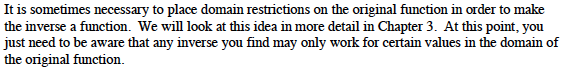### Home > PC > Chapter 1 > Lesson 1.1.3 > Problem1-38

1-38.
1. In the previous problem it appears that g(x) and h(x) are inverses of each other. Test this idea by completing the following compositions. Homework Help ✎

1. g(h(4))

2. h(g(−1))

3. g(h(−2))

4. Did you get the same output as your original input in all of the problems? What happened in part (c)?

5. If you use y = ±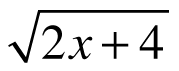as the inverse for h(x), it may seem to resolve this problem. The relation y = ±is not a function. Why is this expression not a function?

It is sometimes necessary to place domain restrictions on the original function in order to make the inverse a function. We will look at this idea in more detail in Chapter 3. At this point, you just need to be aware that any inverse you find may only work for certain values in the domain of the original function.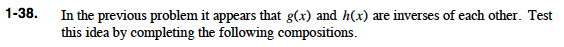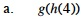$g(x) = \sqrt{2\textit{x}+4}$

$h(x) = \frac{1}{2}\textit{x}^{2} - 2$

$h(4) = \frac{1}{2}4^{2} - 2$

$h(4) = 8 - 2 \Rightarrow 6$

$g(h(4)) = \sqrt{2 \cdot 6 + 4} \Rightarrow 4$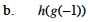Yes, output and input are the same: -1. Show all of your work!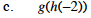No, the output is 2 while the input was -2. Show all of your work!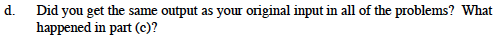It does not have the same output in part(c) because the inverse of the h function is NOT a function.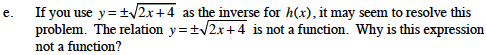There are two outputs for a given input of x. A function can only have one output.